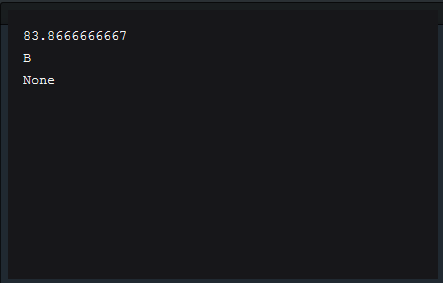0

# Python for beginner - GPA Calculator Sample

### Why learn Python?

Python is a general-purpose, versatile and popular programming language. It's great as a first language because it is concise and easy to read, and it is also a good language to have in any programmer's stack as it can be used for everything from web development to software development and scientific applications.

### Learn by sample code

Beside self-training the basic concept on Python such as: variable, class, data type, ... To give you guys the first look on what Python look like. I wanna show you a traditional sample code which help student calculate their GPA, take a look!

### Show me the code!

A. Create dictionaries

• Create three dictionaries: `lloyd`, `alice`, and `tyler`.
• Give each dictionary the keys "name", "homework", "quizzes", and "tests".
• Now fill out your `lloyd`, `alice` and `tyler` dictionary with the appropriate scores.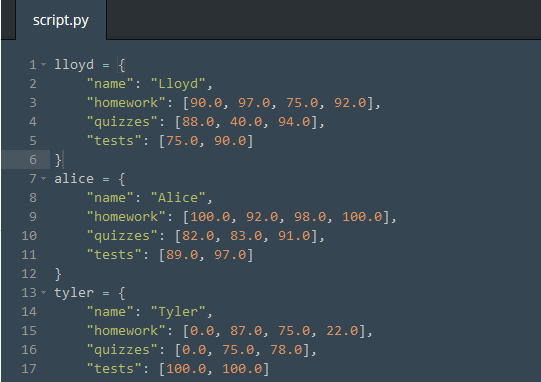• Create a list called students that contains `lloyd`, `alice`, and `tyler`.
• For each `student` in your `students` list, print out that `student`'s data, as follows: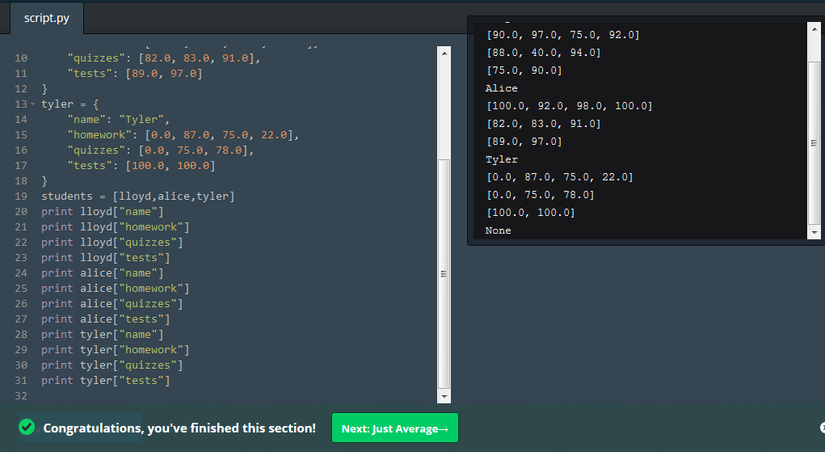B. Create function average

• Define a function called `average` that has one argument, `numbers`.
• Inside that function, call the built-in `sum()` function with the `numbers` list as a parameter. Store the result in a variable called `total`.
• Use `float()` to convert `total` and store the result in `total`.
• Divide `total` by the length of the `numbers` list. Use the built-in `len()` function to calculate that.
• Return that result.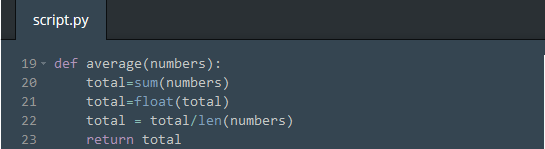C. Create function get_average

• Define a function called `get_average` that takes one argument called `student`.
• Make a variable `homework` that stores the `average()` of `student["homework"]`.
• Repeat step 2 for "quizzes" and "tests".
• Multiply the 3 averages by their weights and return the sum of those three. Homework is 10%, quizzes are 30% and tests are 60%.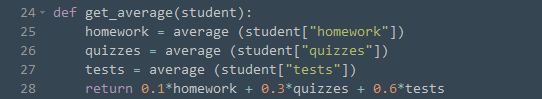• Define a new function called get_letter_grade that has one argument called score. Expect score to be a number.

• Inside your function, test score using a chain of if: / elif: / else: statements, like so:

`````` - If score is 90 or above: return "A"
- Else if score is 80 or above: return "B"
- Else if score is 70 or above: return "C"
- Else if score is 60 or above: return "D"
- Otherwise: return "F"
``````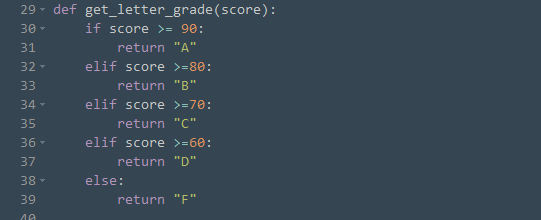E. Create function get_class_average

• Define a function called `get_class_average` that has one argument `students`. You can expect `students` to be a list containing your three students.
• First, make an empty list called `results`.
• For each `student` item in the `class` list, calculate `get_average(student)` and then call `results.append()` with that result.
• Finally, return the result of calling `average()` with results.
• Finally, `print` out the result of calling `get_class_average` with your `students` list. Your `students` should be `[lloyd, alice, tyler]`.
• Then, print the result of `get_letter_grade` for the class's average.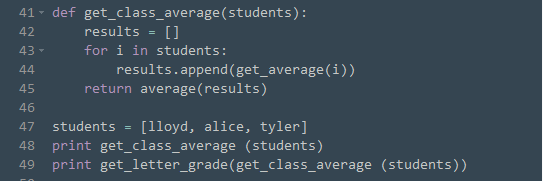### And the result